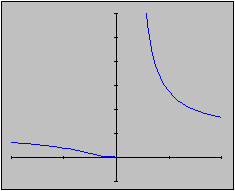#Interactive Real Analysis

Next | Previous | Glossary | Map

## 6.3. Discontinuous Functions

### Examples 6.3.8(a):

What kind of discontinuity does the function f(x) = exp(1/x) have at x = 0 ?Your browser can not handle Java applets f(x) = exp(1/x)

As x approaches zero from the right, 1/x approaches positive infinity. Therefore, the limit of f(x) as x approaches zero from the right is positive infinity.

As x approaches zero from the left, 1/x approaches negative infinity. Therefore, the limit of f(x) as x approaches zero from the left is zero.

Since the right-handed limit fails to exist, the function has an essential discontinuity at zero.

Next | Previous | Glossary | Map